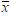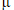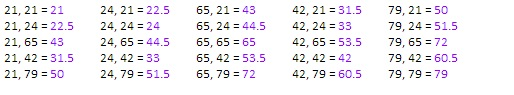PURCHASE A DIGITAL COPY PURCHASE A HARD COPY Lesson 1 Introduction to Statistical Research Methods Lesson 2 Visualizing Data Lesson 3 Central Tendency Lesson 4 Variability Lesson 5 Standardizing Lesson 6 Normal Distribution Lesson 7 Sampling Distributions Lesson 8 Estimation Lesson 9 Hypothesis Testing Lesson 10 t-Tests for Dependent Samples Lesson 11 t-Tests for Independent Samples Lesson 12 Intro to One-Way ANOVA Lesson 13 One-Way ANOVA: Test significance of differences Lesson 14 Correlation Lesson 15 Linear Regression Lesson 16 Chi-Squared Tests Afterward Index

Now you’ve seen that by knowing the mean and standard deviation of a normally distributed population, we can describe how a value compares to others in the population in terms of its percentile. And, we can also deduce the percent of values in-between, less than, or greater than any values.

In this lesson, we’ll apply this same logic to samples. How can we decide if a sample of values is typical or atypical? Let’s start with a simple example.

We have this population (for simplicity, N = 5):

21       24       65       42       79

Now let’s say we randomly select a sample of size n = 2, and we get 21 and 24.

21       24       65       42       79

How can we decide if this sample is typical of the population? You may guess that we can compare the mean of this sample to the mean of the population. That’s a start.= 22.5= 46.2

The mean of the sample looks very different from the mean of the population, but how different? Well, let’s look at the means we could get from all other possible samples of size n=2.To continue learning, purchase Street-Smart Stats: A Friendly Introduction to Statistical Research Methods.